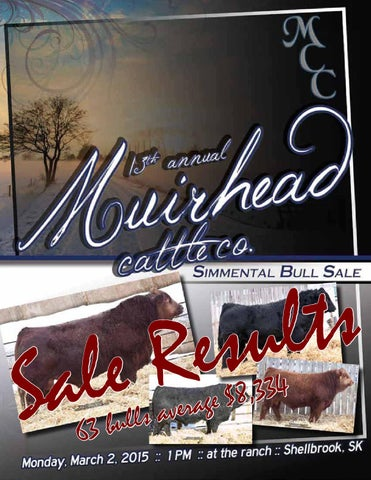l a S

s t l u s e R e

63

r e v a bulls

4 3 3 , 8 $e g a$11,000

$10,000$10,500

$8,000$9,000

$8,000$8,500

$8,250$9,000

out of sale

$14,000$14,500

$9,000$9,500

$7,750$9,500

$9,500$7,700

$8,000$8,000

out of sale

$9,500$11,000

$11,000$11,500

$10,500$9,000

$8,500$6,500

$8,500$10,500

$9,000$7,750

$11,750$9,500

$9,000$8,500

$5,500$6,500

$7,000$8,500

$6,750$6,500

$6,250$7,500

$5,750$8,500

$9,000 out of sale$7,000

$6,000$7,750

$13,000$10,250

$4,100$6,000

$8,500$5,000

$6,000$8,000

$8,250$5,000

$4,250$6,000

$5,500$5,000